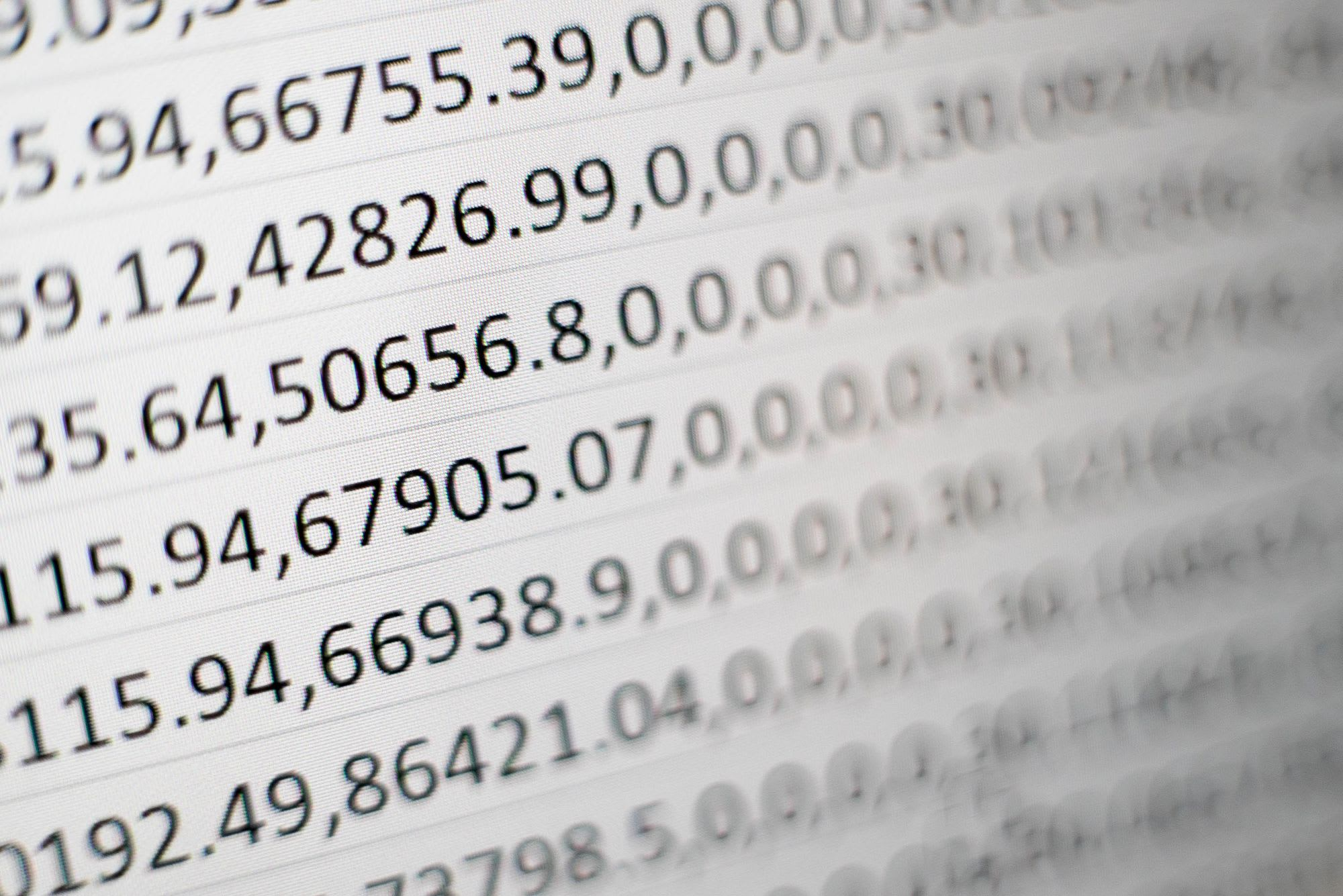deep learning

# Data generator using a Sequence in Tensorflow 2

A simple example in how to use a custom data generator using TF 2

First, let me explain the context: I executed a convolutional neural network (CNN) using 2D grayscale images (extracted from MRIs). Then, my poor Nvidia GeForce GTX 1070 with 8GB of RAM is not enough to load the dataset 🥺. One possible solution is to buy a new graphics card! 🤑. However, that is not an option for me.

Another option is handling how to load the data into a limited memory of a graphics card: using small blocks of memory that fit into the memory to feed the network right away. Sometimes, these blocks are usually known as batches or chunks. Then, I discovered the Sequence object in Tensorflow.

I forgot to mention that this post is coded for Tensorflow 2 using Python 3. Now, let me explain my solution using the Sequence object 🤓

### Solution

Only focus on the training stage, only considering training and validation data, and ignoring the test/evaluation data. Images have a size of 64x64 pixels with one single channel. The data is loaded into the source object, and the function `load_data` splits that data into training and validation. In this case, the splitting is 80% for training, and 20% for validation is made.

``````keras.backend.set_image_data_format('channels_first')
# ...
# ...
model = get_model(hyperparameters)
``````

Also, the CNN model was constructed and compiled using a function called `get_model`. That function creates the models using the layer structure for your CNN architecture (e.g. sequential model).

Notice that training data and validation data are stored into the NumPy arrays `(x_train, y_train)` and `(x_val, y_val)` respectively. Just for simplicity, I assumed that train and validation data have the following shapes:

``````# x_train.shape is (800, 64, 64, 1)
# x_val shape is (200, 64, 64, 1)
``````

The habitual way to train the network using Tensorflow is as follows:

``````model.fit(x=x_train, y=y_train)
``````

However, this throws an error about the capacity to store data into the graphics card's memory 🤯. Ok, then I must split the data in some way using the Sequence class. This object is handled for fitting to a sequence of data like dataset. The significant thing is the Sequence could be extended, and it must implement three methods:

1. `__init__`: initializing the dataset / variables
2. `__len__`: returning the legth of the dataset
3. `__getitem__`: extracting an item from dataset

Remember you have to implement these methods into a class that extends the Sequence class. It is possible to create a complex dataset process for extraction. For instance, you can implement functions as `on_epoch_end` which triggered once at the very beginning as well as at the end of each epoch.

To enter the Keras code, let me define a couple of callbacks 😎:

``````from tensorflow_addons.tfa.callbacks import TQDMProgressBar
from tensorflow.keras.callbacks import EarlyStopping

tqdm_callback = TQDMProgressBar()
early_callback = EarlyStopping(monitor='val_acc',
verbose=1,
patience=10,
mode='max',
restore_best_weights=True)
``````

The `tqdm_callback` is a progress bar during training (see TQDM Progress Bar), and `early_callback` is a way to early stopping the training according a monitor value, in this case the validation accuracy value (see EarlyStopping).

Then, the idea is using a data generator in the `fit` function (in previous Tensorflow's versions, the function was `fit_generator`). Generators are functions which at the end of them use the command `yield` instead of the `return` keyword. Remember that `yield` saves the state of the function and continues from there successively is called. In this way, the `yield` returns an object whose value can be accessed by employing the next method.

Using a custom class called DataGenerator inheriting the tensorflow.keras.utils.Sequence class, we need to implement the three functions mentioned:

``````from tensorflow.keras.utils import Sequence
from math import ceil

class DataGenerator(Sequence):

def __init__(self, x_set, y_set, batch_size):
self.x, self.y = x_set, y_set
self.batch_size = batch_size

def __len__(self):
return ceil(len(self.x) / self.batch_size)

def __getitem__(self, idx):
end = min(self.x.shape, (idx + 1)*batch_size)
return self.x[idx*batch_size:end], self.y[idx*batch_size:end]
``````

Now, let me explain the previous code: `x_set`, `y_set` and `batch_size` are the required values for the class. Focus on the `__len__` function where computes the size of the batch, chunk or small block to be passed to the graphics card's memory using the Python generator. For the function, it is possible using the `self.y` instead `self.x`, just to select one.

The `__getitem__` is the core of the class where the idea is to determine where data should be extracted, from the beginning to its end. Notice the parameter `idx` which represents how many blocks should be used. For instance, if `batch_size` is equal to 100, then the `__len__` function should return the value of 8 and the `idx` takes values from 0 to 7. The slicing property is utilized to select the start:end of the dataset 👻.

Once we already produced the class for the generator, we can invoke the `fit` function as follows:

``````batch_size = 256
epochs = 100

training_generator = DataGenerator(x_train, y_train, batch_size)

history = model.fit(x=training_generator,
steps_per_epoch=x_train.shape//batch_size,
validation_data=(x_val, y_val),
epochs=epochs,
verbose=0,
use_multiprocessing=True,
workers=8,
callbacks=[tqdm_callback, early_callback])
``````

I know there are furthermore about sequences, generators, the partition of data, and more; however, in this post just points a way that works for me and I hope that could be valuable for anyone. You can use this as a guide on your TensorFlow code! 😉 Remember that structure depends entirely on your problem and your data structures defined on your code...then, good luck human! 👽